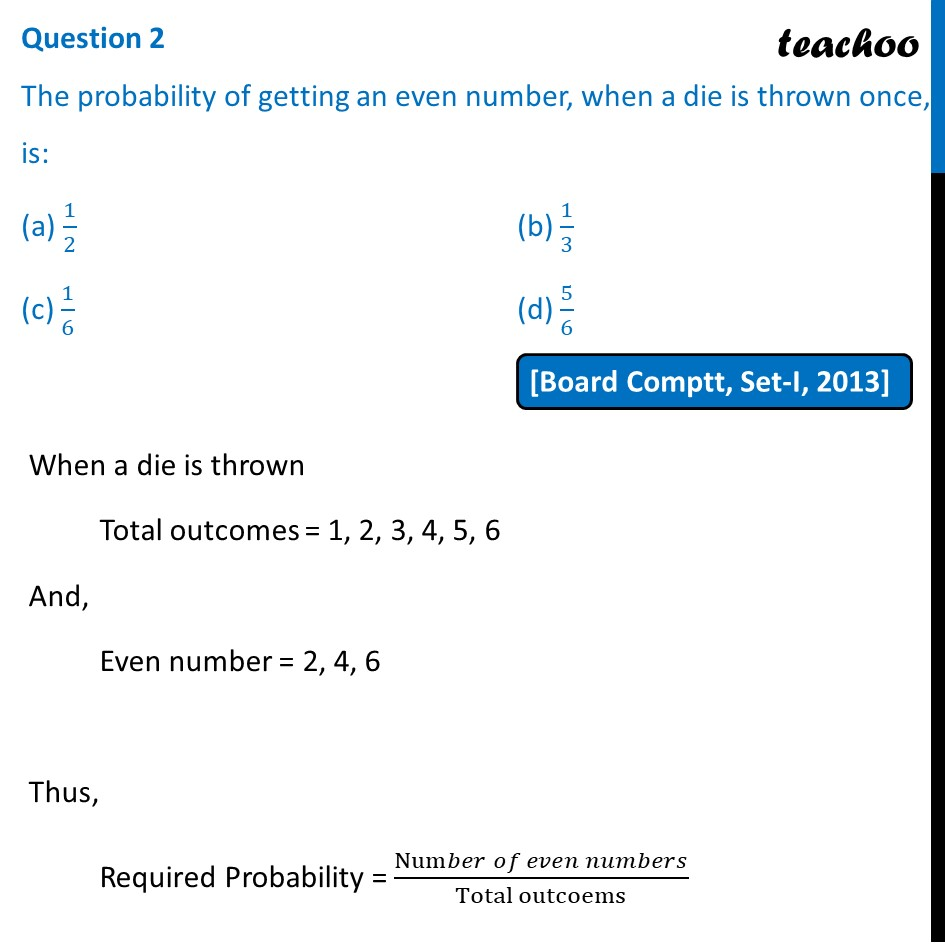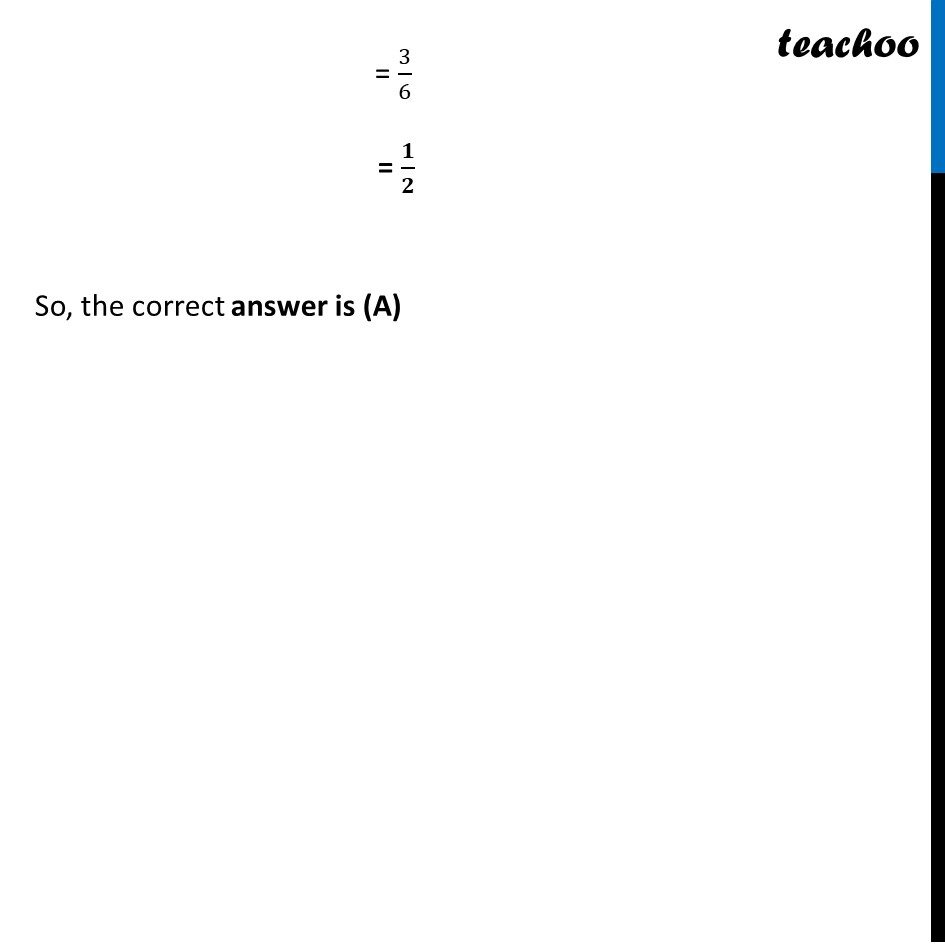Past Year MCQ

Chapter 14 Class 10 Probability
Serial order wise

## (c) 1/6                                                          (d) 5/6Learn in your speed, with individual attention - Teachoo Maths 1-on-1 Class

### Transcript

Question 2 The probability of getting an even number, when a die is thrown once, is: (a) 1/2 (b) 1/3 (c) 1/6 (d) 5/6 When a die is thrown Total outcomes = 1, 2, 3, 4, 5, 6 And, Even number = 2, 4, 6 Thus, Required Probability = (Num𝑏𝑒𝑟 𝑜𝑓 𝑒𝑣𝑒𝑛 𝑛𝑢𝑚𝑏𝑒𝑟𝑠)/(Total outcoems) = 3/6 = 𝟏/𝟐 So, the correct answer is (A)